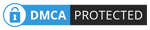# How to Convert Your CGPA to Percentage

SHARE

While it is common practice to calculate GPA and CGPA, there are times when you’ll be required to present your result in percentage. Some educational institutions look for percentages of your score rather than Cumulative Grade Point Average. In such a situation, knowing how to convert your CGPA to percentage can be useful.

In most climes, the Cumulative Grade Point Average is calculated on a 4, 5, 7 and 10 point grading scale. The grading system helps teachers and students establish the levels of achievement by either percentages or alphabetical symbols that indicate grading such as A, B, C and D.

Having said that, in this article, you’ll learn how to convert your CGPA to percentage. For the purpose of this article, we’ll be using the 4 and 5 point scale in our conversion.

Furthermore, we’ll also look at common terminologies and how to calculate the Cumulative Grade Point Average (CGPA) for both 4 points and 5 points grading scale.

## Common Terminologies Used in Calculating CGPA

1. CCT– Cumulative Credits Taken
2. CCP– Cumulative Credits Passed
4. FGPA– Final Grade Point Average

CCT CALCULATION

• This is the sum of all credit hours in courses taken.
• It is usually based on course codes and not course titles
• It is calculated once.

CCP CALCULATION

• This is the sum of all credit hours of passed courses
• Passed grades do not include grades E and F (for the 4 point scale)
• Grades A to D are calculated here

 Letter Grade Marks Point A 80 – 100 4.0 B+ 75 – 79 3.5 B 70 – 74 3.0 C+ 65 – 69 2.5 C 60 – 64 2.0 D+ 55 – 59 1.5 D 50 – 54 1.0 F 0 – 49 0

 Letter Grade Marks Point A 70 – 100 5 B 60 – 69 4 C 50 – 59 3 D 45 – 50 2 F 0 – 44 1

### CALCULATION OF GPA (4 Point System)

 Course Code Credit Grade Grade Point Grade Point Total SOCI121 3 A 4 12 SREL111 3 A 4 12 UGRC120 3 C+ 2.5 7.5 GST113 3 C 2.0 6.0 BOT121 3 B+ 3.5 10.5 MTH118 4 B 3.0 12

Total GPT=60

• Total Credits = 19
• GPA = 60/19

GPA = 3.1

### CALCULATION OF GPA (5 Point System)

 Course Code Credit Grade Grade Point Grade Point Total SOCI121 3 A 5 15 SREL111 3 A 5 15 UGRC120 3 C 3 9 GST113 3 C 3 9 BOT121 3 B 4 12 MTH118 4 B 4 16

Total GPT=76

• Total Credits = 19
• GPA = 76/19

GPA = 4.0

## CALCULATION OF CGPA

• This is for the total semesters a student has taken examinations in the university
• All levels carry equal weight
• CGPA= Total GPTs of all courses taken/ Total Number of Credits of all courses taken

Assuming you’ve taken the examination in Level 400 second semester, the GPTs and Credits will be calculated from L100 first and second semesters, L200 first and second semesters, L300 first and second semesters and L400 first and second semesters.

## How to Convert Your CGPA to Percentage

As earlier mentioned, you’ll learn how to convert your CGPA to percentage with this formula below.

 If Formula CGPA>=3.63 (cgpa-0.3)/0.037 CGPA>=3.25 (cgpa-0.29)/0.037 CGPA>=2.88 (cgpa-0.36)/0.036 CGPA>=1.8 (cgpa+1.65)/0.069 CGPA>=1 (cgpa+2.16)/0.079 CGPA>0 (cgpa/0.0248)

From the example of 4 point grading above, assuming final CGPA on a 4 point scale is 3.1, converting to percentage; we use this formula (CGPA-0.36)/0.036 because CGPA >=2.88

(3.1-0.36)/0.036 = 76.1%